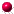# GMT xyplots examplesExample B1: Number of earthquakes per year: dotsExample B2: Number of earthquakes per year: dots and curveExample B3: Number of earthquakes per year: vertical barsExample B4: Schematic diagram (no explanations)Example B5: Geological Cross-section (no explanations)Example B6: WigglesExample B7: 3-D plot